Courses

# Signals And Systems & Microprocessors (EE & EC GATE) - MCQ Test 1

## 15 Questions MCQ Test Mock Tests Electronics & Communication Engineering GATE 2020 | Signals And Systems & Microprocessors (EE & EC GATE) - MCQ Test 1

Description
This mock test of Signals And Systems & Microprocessors (EE & EC GATE) - MCQ Test 1 for Electronics and Communication Engineering (ECE) helps you for every Electronics and Communication Engineering (ECE) entrance exam. This contains 15 Multiple Choice Questions for Electronics and Communication Engineering (ECE) Signals And Systems & Microprocessors (EE & EC GATE) - MCQ Test 1 (mcq) to study with solutions a complete question bank. The solved questions answers in this Signals And Systems & Microprocessors (EE & EC GATE) - MCQ Test 1 quiz give you a good mix of easy questions and tough questions. Electronics and Communication Engineering (ECE) students definitely take this Signals And Systems & Microprocessors (EE & EC GATE) - MCQ Test 1 exercise for a better result in the exam. You can find other Signals And Systems & Microprocessors (EE & EC GATE) - MCQ Test 1 extra questions, long questions & short questions for Electronics and Communication Engineering (ECE) on EduRev as well by searching above.
QUESTION: 1

### In a continuous time system x(t) and y(t) denotes the input and output respectively then which of the following corresponds to a casual system?

Solution: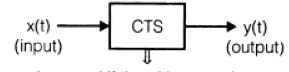is casual if the y(I) at any the
depends only on presarit of past or both
present and past values of xit).

So, here y(t) = + 4) x(t -1) is only a casual system.

QUESTION: 2

### If f(x) =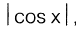, for Fourier series of x(t) in the interval (-π, π), the value of ao and a1 respectively is

Solution:

Since,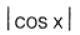is an even function_

So.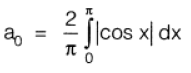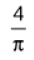and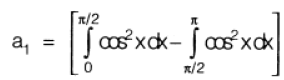= 0

*Answer can only contain numeric values
QUESTION: 3

### The fundamental period of signal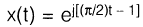Solution: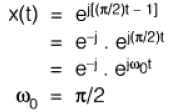Here,

So, fundamental period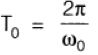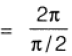= 0

QUESTION: 4

The Laplace transform of a continuous-time signal x(t) is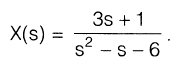If the Fourier transform of this signal exists, then x(t) is

Solution: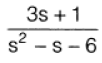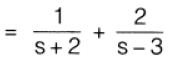If the Fourier transform of the signal exiss, then ROC must be-2 < Re(s) < 3. Therefore, x(t) e 2t u(t) — 2e31 u(-t).

QUESTION: 5

The ROC for the Laplace transform of signal

x(t) = e-2t {u(t) — u(t — 5)] is given by

Solution: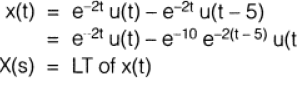∴​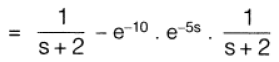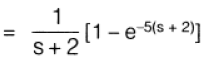for, Re(s) > -2

QUESTION: 6

Under what condition the energy of signal g1(t) and g2(t) equals to E91 + Eg2

Solution: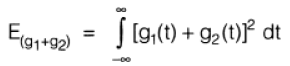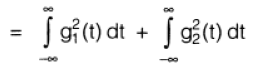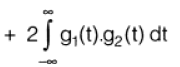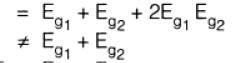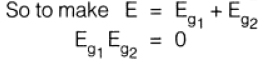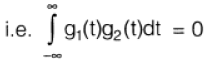QUESTION: 7

The signal V(t) = cos 5 πt + 0.5 cos 10 πt,is instantaneously sampled. The interval between the samples is 'TS'. Then the maximum allowable value for 'Ts' is

Solution:

(c)

Frequencies in the given signals are 2 πf = 5 π and 2 πf = 10 π i.e. 2.5 Hz and 5 Hz.

∴. Sampling frequency

= fs

= 2 fm

= 10 Hz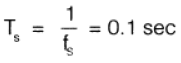QUESTION: 8

Inverse Laplace transform of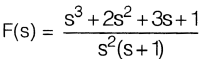Solution: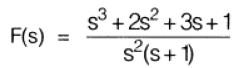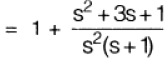Solving by use of partial fraction;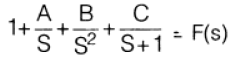We get,          A = 2,

B = 1

and      and      C = --1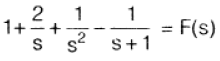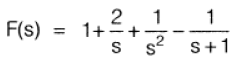∴  f(t)  Inverse laplace of F(s)

f(t)  =  δ(t) + 2 + t - e-t

QUESTION: 9

Match List-I (Type of Addressing Modes)

with List-II (Instructions set) and select the correct answer using the codes given below the lists:

List-I

3. Register indirect mode

List-II

1. MOV A, M

2. MOV A, B

3. CMA

4. STA 2700H

Codes: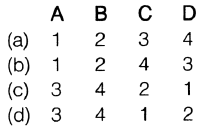Solution:
QUESTION: 10

Consider the following statements:

1. Programme Status Word (PSW) = Accumulator + Flag Register + Program Counter
2. Program counter is a 16-bit register.
3. Stack pointer operates as last in last out.
4. "TRAP" is both level and edge sensitive. Which of the statements are false?
Solution:

PSW = Accumulator Flag Registor only

and SP operates as LIFO (Last in First out).

QUESTION: 11

In priority orders, the interrupts in microprocessor are as

Solution:

In priority order, interrupts are as,

TRAP > RST7 .5 > RST6_5 HST5.5> INTR.

QUESTION: 12

A memory chip of size 4 k byte has starting location 2000H. The last location of memory chip is given by

Solution:

Total number of memory locations

= 22 x 210

212

Since in itia memory location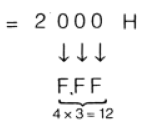QUESTION: 13

The 8085 assembly language instruction that stores the content of H and L register into the memory locations 2050H and 2051H, respectively is

Solution:
QUESTION: 14

The following program is run on an 8085 microprocessor:

(in hex)

3000                                LXI SP, 4000

3003                                 PUSH H

3004                                 PUSH D

3005                                  CALL 3050

3008                                  POP H

3009                                   HLT

At the completion of execution of the program, the program counter of 8085 and stack pointer contains

Solution:

(c)

3000            SP → 4 4000

3003             SP → SP decremented                        by 2      i_e_ 3FFE

3004             SP SP decremented                        by 2  3FFC

3005          PC 3050

SP  3FFC

PC 3050

QUESTION: 15

15 8 memory chips of 64 x 4 bit size have address buses connected together. The resultant memory is

Solution: